Reach Us+1-217-403-9671
Detection of Camouflaged People | OMICS International
International Journal of Sensor Networks and Data Communications

# Detection of Camouflaged People

Bento NA1, Silva JS1* and Dias JB2

1CINAMIL and DCTE, Military Academy, Lisbon, Portugal

2Electrical and Computer Engineering Department, Instituto Superior Técnico, Lisbon, Portugal

*Corresponding Author:
Silva JS
CINAMIL and DCTE
Military Academy, Lisbon, Portugal
Tel: +351214985660
E-mail: [email protected]

Received date: June 10, 2016; Accepted date: July 02, 2016; Published date: July 11, 2016

Citation: Bento Na, Silva JS, Dias JB (2016) Detection of Camouflaged People. Sensor Netw Data Commun 5:143. doi: 10.4172/2090-4886.1000143

Copyright: © 2016 Bento NA, et al. This is an open-access article distributed under the terms of the Creative Commons Attribution License, which permits unrestricted use, distribution, and reproduction in any medium, provided the original author and source are credited.

Visit for more related articles at International Journal of Sensor Networks and Data Communications

#### Abstract

The use of thermal imaging is a benefit for the military people. Due to their advantages, it has a large number of applications, including the detection of camouflaged people. For better results, the thermal information can be merged with the color information which allows a greater de-tail, resulting in a higher degree of security. The present work implemented as pixel level image fusion methods: Principal Components Analysis, Laplacian Pyramid, and Discrete Wavelet Transform. A qualitative analysis concluded that the method which performs better is the one that uses Wavelets, followed by the Laplacian Pyramid and finally the PCA. A quantitative analysis was made using the metrics: Standard Deviation, Entropy, Spatial Frequency, Mutual Information, Fusion Quality Index and Structural Similarity Index. The values obtained support the conclusions extracted from the qualitative analysis.

#### Keywords

Laplacian pyramid; Pixel level image fusion; Metrics; Principal component analysis; Security; Wavelets

#### Introduction

Nowadays the majority of surveillance systems use detection systems through color, however these systems are highly limited by luminosity. The use of infrared cameras allows to capture the thermal image of an object, with a benefit for the military due to its ability to daytime and nighttime use, as well under different weather conditions . In this context, these images can be used to detect camouflaged people.

To enhance the results of people detection, the color information can be combined with thermal information. While the color images give a visual context to objects, thermal images give information about objects with high temperature. The fusion of both images gives a better visual perception of the scene and it allows a better detection of people. This work aims to implement pixel level image fusion methods to detect camouflaged people.

#### Background

Over the years, various image fusion techniques have been proposed to cope with its growing demand, as there are several areas that benefit from this process, including: Medical [2,3], military , surveillance and navigation. Naidu and Raol  make a comparison of pixel level fusion methods using Wavelets and Principal Component Analysis (PCA). Regarding to Wavelets, the fusion rule used was the simple average for the approximation coefficients and detail coefficients with the largest absolute value, and tested five levels of decomposition. In their study, some metrics were implemented, with and without a reference image to evaluate the performance of image fusion algorithms. Metrics such as Standard Deviation, Entropy, Cross- Entropy and Spatial Frequency were considered appropriate when there is no reference image. They concluded that the Image fusion using Wavelets with a greater degree of decomposition has better performance.

Zheng  makes a comparison of multi-scale pixel level fusion algorithms, such as: Different Pyramids, Discrete Wavelet Transform (DWT) and Iterative DWT. Zheng proposed the Advanced DWT algorithm for image fusion. In this algorithm, the approximation coefficients at the largest scale of the input image are fused by applying the PCA to the absolute values of these coefficients. It was subsequently optimized with an iterative procedure using the fusion metrics: Image Quality Index and Error Rate of the Spatial Frequency. The author used three pairs of images; the assessment of the fused images was qualitatively and quantitatively made. As quantitative metrics, he used the Entropy and Spatial Frequency. The quantitative results show that the iterative algorithms have better performances, followed by Laplacian Pyramid and finally the DWT.

Sadhasivam et al.  implemented a PCA using the maximum principle. Since the results obtained from the traditional PCA show a low performance when compared with other hybrid algorithms, the authors implemented an algorithm that uses the DWT in conjunction with PCA. The low-frequency coefficient is chosen according to the maximum rule, and PCA is to be applied to the high frequency coefficients to determine their weights for the fusion. The final image is obtained by adding the low and high frequency images. They used three sets of images and the performance of this algorithm was measured by Entropy, Mutual Information and the Structural Similarity Index. While Entropy presents similar values for the compared methods, Structural Similarity and the Mutual Information in the fused image have better results for the proposed method.

Zheng et al.  develop an algorithm that incorporates the PCA in DWT. The PCA is applied to the approximation coefficients, whereas the detail coefficients are chosen in accordance with the largest absolute value. The proposed algorithm is compared to other fusion techniques using Entropy, the Spatial Frequency and Image Quality Index, in cases where there is no reference image.

#### Methods

In this section, it is done a brief explanation of the methods used in this work: PCA, Laplacian Pyramid and Wavelets, and the metrics used for quantitative analysis.

Principal component analysis

The PCA involves a mathematical procedure that transforms a number of correlated variables into a number of uncorrelated variables called principal components.

The image fusion is achieved through a weighted average of the images to be fused. The weights for each input image are obtained from the eigenvectors corresponding to the highest eigenvalue of covariance matrices for each input image. The fused image is given by :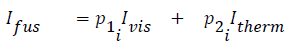where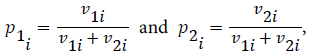computed from the covariance matrix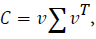where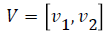and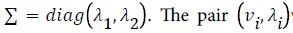with i = 1, 2 corresponds to the pair eigenvector/eigenvalue of the C where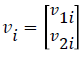.

Laplacian pyramid

This method decomposes the input image in sub-images with different spatial resolutions. A fusion rule is used to construct a representation of a fused pyramid. The lowest level of the pyramid is built from the original input image and each of the other levels is built recursively from its lowest level by applying four steps [8-9]: Blurring, subsampling, expansion by interpolation and differentiation.

There are two standard operations: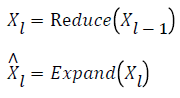The Laplacian Pyramid is applied to decompose the input images at N levels: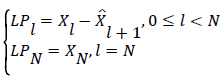The reconstruction of the image from the Laplacian Pyramid is the inverse process of decomposition and in the reverse direction, from the top level to the bottom level: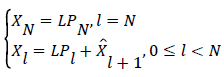Having two images to fuse, IVis and Itherm, the construction of the pyramid is made for each image individually.

Wavelets

Wavelets theory has been widely used in image processing and provides a multi-resolution decomposition of an image. The input images are decomposed into approximation and detail coefficients at a certain level by using the DWT. Then approximation and detail coefficients are combined using the fusion rule producing the fused image by applying the inverse DWT: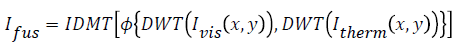The fusion rules used in this work are:

The maximum approximation coefficient at the largest scale and the largest absolute value of the detail coefficients in each transformed scale; the mean of approximation coefficients at the largest scale and the largest absolute value of the detail coefficients in each transformed scale.

Quantitative evaluation

It is important to make a quantitative assessment such that fusion algorithms can be analyzed and compared objectively. The metrics used are:

Standard deviation: The Standard Deviation measures the contrast of the fused image, defined as: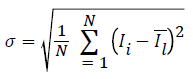Where Ii is a column vector of N observations and Il is the mean of that same vector. An image with high contrast has a high standard deviation value .

Entropy: The Entropy can measure the information content of an image, but it can’t distinguish the noise information. The entropy is given by :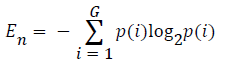where G is the number of gray levels in the histogram of the image, typically between 0 and 255, and (i) is the normalized frequency of occurrence of each gray level. An image with high information content will have high entropy.

Spatial frequency: The Spatial Frequency indicates the overall activity level of an image, defined as :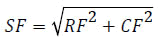where RF corresponds to row frequency and CF to columns frequency. The frequencies of lines and columns are given by: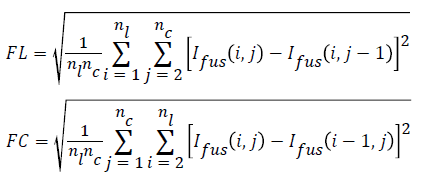Where nl is the number of lines and nc the number of columns of an image. A high value for the spatial frequency indicates a high overall activity. The higher its value the more information has the fused image.

Mutual information: This metric measures the degree of dependence between two images. It is calculated by setting the joint histogram of input images Ivis, Itherm, and the fused image Ifus. The mutual information between the input images and the fused image  is given by: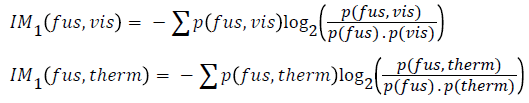where (fus, vis) p (fus, threm) are the joint histograms of input images and fused image. The efficiency of the fusion algorithm is determined by the IM metric which is defined by: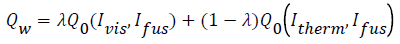A greater dependence, that is, a larger value means better quality.

Fusion quality index: The Fusion Quality Index of an image measures the similarity between the fused image and both input images and it is defined by: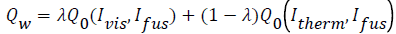where Q0 is the overall quality index, and λ is a local weight that indicates the relative importance of the input image compared to the fused image. The overall quality index Q0 of two images is defined by: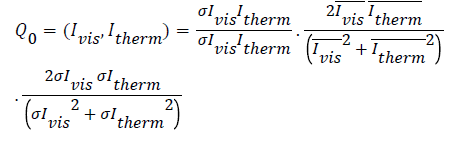This metric can assume values between 0 and 1, where 1 corresponds to a better quality of the fused image.

Structural similarity index: The Structural Similarity Index has been used to indicate the similarity of the information structure between two images and is defined by :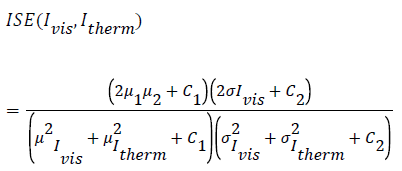where μ is the mean of the intensity of the image, σ is the standard deviation of the image and C1 and C2 are constants.

The total Structural Similarity Index is given by: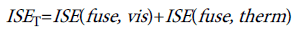(18)

A larger value indicates that the information present in each input image is also present in the fused image.

#### Results

In this section, the results are presented from each of the methods used, followed by a qualitative and quantitative analysis.

Data set

The images used for this work were acquired with a FLIR T440bx camera and have 320 × 240 pixels, obtained in two Military Academy exercises. For tests, 20 images were selected, which represents camouflaged people in different scenes and light conditions. The algorithms were implemented using Matlab.

Qualitative analysis

In order to compare the three implemented methods, the third variant of PCA, Laplacian Pyramid with four levels of decomposition and Wavelets with one level of decomposition using the 1st fusion rule were applied to 20 pair of images producing 20 fused images.

In this first example, Figure 1, the subjective quality of the three images is very similar, second image has a lower contrast visible in the silhouette of people in the tree shade, relatively to the other two.

Figure 1: a) Third variant of PCA; b) Laplacian Pyramid with four levels of decomposition; c) Wavelet with one level of decomposition and 1st fusion rule.

In the second example, Figure 2, the quality of the first two images is similar, and in the third image the person is most prominent in relation to the other two, being preferred for that reason. The detail in the three images is identical.

Figure 2: a) Third variant of PCA; b) Laplacian Pyramid with four levels of decomposition; c) Wavelet with one level of decomposition and 1st fusion rule.

In the third example, Figure 3, a person is behind bushes, which contributes to the lack detail because the bushes are dense, and the input images appear to be a stain. The third image is where you can observe increased intensity of the thermal component, however the second image is the one that appears to have a better quality because it has a balance between detail and thermal component; despite being able to identify the person you can also see that the darker parts of the image have different shades (unlike what happens in the third image).

Figure 3: a) Third variant of PCA; b) Laplacian Pyramid with four levels of decomposition; c) Wavelet with one level of decomposition and 1st fusion rule.

The method that seems to have better results is one that uses Wavelets, followed by the method using the Laplacian Pyramid. One of the factors that contributed to the poor results produced by the method using the PCA is the fact that when both of the principal component values are near to 0.5, the fusion resembles the fusion through the simple average which produces a low contrast of features.

On the other hand, the method using the Wavelets is the one with better results because it is the one that does the fusion of the various image components (approximation, horizontal, vertical and diagonal coefficients) in line with the established fusion rules to be the best for the intended purpose, which is to detect the camouflaged people.

Quantitative analysis

In this section is performed a quantitative assessment for comparison of the performance of each method used. The results are presented in boxplot charts.

In these figures, an objective comparison is made, wherein each bin corresponds to a method. The methods are designated as follows: PCA1, PCA2 and PCA3 (three variants of the PCA); LP02 and LP04 (Laplacian Pyramid with 2 and 4 levels); WV11 and WV12 (wavelet with 1 level of decomposition with 1st or 2nd fusion rule); WV21 and WV22 (wavelet with 2 levels of decomposition with 1st or 2nd fusion rule).

Spatial frequency: The Spatial Frequency indicates the overall activity level of an image, that is, the greater its value more information has the image, except in cases where there is the introduction of noise in the fusion process.

Spatial Frequency of the images obtained with the method using the PCA has values close to zero. The method which achieves better results is the Laplacian Pyramid with four levels of decomposition, because it preserves the outlines of images and thus contributes with a greater detail to the fused image, followed by Wavelets with two levels of decomposition, which have a performance better than those with one decomposition level, contrary to what was expected (Figure 4).

Mutual information: The value of the Mutual Information is the sum of the Mutual Information of each input image with the fused image, so the greater its value the greater is the dependence of the input images and the fused image.

From Figure 5, the best results were obtained when using wavelets with the 1st fusion rule, both with one and two levels of decomposition; results with PCA are the worst. The highest values depend on the fact that the pixels with the highest intensity being selected, information transfer is increased. On the other hand, in the Laplacian Pyramid and Wavelets with the 2nd fusion rule, the average of the pixels and therefore the mutual information values are lower.

Fusion quality index: The Fusion Quality Index measures the similarity between the fused image and the input images. It takes values between zero and one, and the higher the similarity the nearest to one is the value.

The best results are obtained when using the method based on PCA, followed by the method that uses the Laplacian Pyramid (Figure 6).

Contrary to what has been observed in the previous metrics, methods based on PCA achieves the highest values. This can be explained by the fact that in this method, a weighted average of the input images is made for the fused image and thus both input images contribute to the final image in the same way. That is, while in methods that use Wavelets there are coefficients that are selected from only one of the input images (having detail coefficients of one of the input images that do not contribute to the fused image), in methods based on PCA that does not happen. This metric is considered to be the most reliable for fused images that do not have reference image ; however, the results for PCA methods are misleading.

#### Conclusions

This work arises from the need to implement a method enabling the detection of camouflage people while they are dissimulated in their environment, making their camouflage inefficient. The objective is to implement pixel level image fusion methods, which intend to merge visible images with thermal images getting a richer fused image. There were implemented three methods: PCA, Laplacian Pyramid and Wavelets.

It was done a qualitative analysis of the fused images based on four sets of three images (third variant of the PCA, Laplacian Pyramid with four levels and Wavelets with one decomposition level and the 1st fusion rule). From this analysis, it is concluded that the method which achieves better results uses Wavelets followed by the method using Laplacian Pyramid and finally using the PCA.

A quantitative analysis was performed using six performance metrics: Standard Deviation, Entropy, Spatial Frequency, Mutual Information, Fusion Quality Index and Structural Similarity Index. This analysis was done based on twenty images obtained for each of the methods and their variants, making a total of nine methods. The results obtained for each metric are shown in boxplot charts, which provide a good insight of the set of results. By observing the boxplot charts obtained, the values for the three variants of the PCA method are those that stand out the negative, because they are well below of those obtained for the remaining methods, which only with a visual inspection was not noticeable.

The values obtained for the methods using the Laplacian Pyramid and Wavelets are within the ranges obtained by other authors, and support the conclusions drawn from the qualitative analysis.

Of the six performance metrics implemented, it is concluded that the Standard Deviation, Entropy and Spatial Frequency metrics are suitable for making a relative comparison among implemented methods (for measuring the quality of the fused image), however, these metrics do not take into account the relationship between the fused image and the input images. Thus, the Mutual Information, Fusion Quality Index and the Structural Similarity Index take into account this relationship and therefore are considered the most appropriate metrics to measure the quality of image fusion.

Comparing both qualitative and quantitative results, it is noted that the methods considered as the best in qualitative assessment are in fact the best quantitative results, that is, the method that uses the Wavelets with one decomposition level with the 1st fusion rule and the method using the Laplacian Pyramid with four levels.

#### References

Select your language of interest to view the total content in your interested language

### Recommended Conferences

• 2nd International Conference on Artificial Intelligence, Robotics & IoT
May 09-10, 2019 Amsterdam, Netherlands
• 5th Global Summit and Expo on Multimedia, Blockchain & Artificial Intelligence Technology
June 17-18, 2019 Berlin, Germany
• 5th International Conference on Wireless,Telecommunication & IoT
June 20-21, 2019 Rome, Italy

### Article Usage

• Total views: 9413
• [From(publication date):
September-2016 - Mar 25, 2019]
• Breakdown by view type
• HTML page views : 9244

## Post your commentCan't read the image? click here to refresh
###### Peer Reviewed Journals

Make the best use of Scientific Research and information from our 700 + peer reviewed, Open Access Journals

International Conferences 2019-20

Meet Inspiring Speakers and Experts at our 3000+ Global Annual Meetings

Top